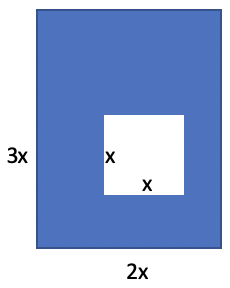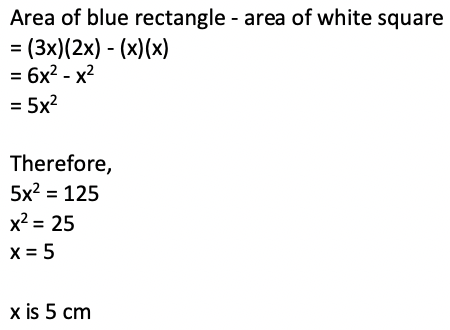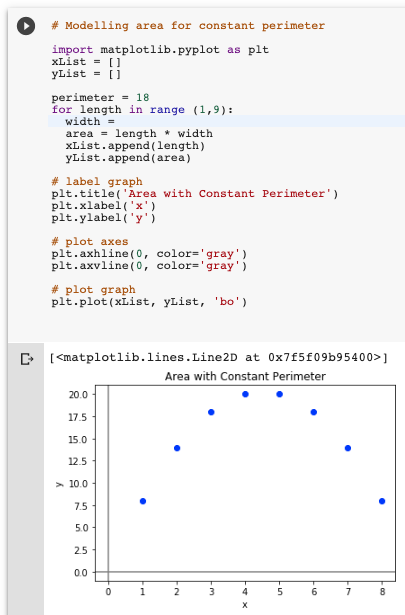# Area and algebraic expressions

### QUESTIONThe area of the blue rectangle, without the white square, is 125 sq. cm.

What is the side length (x) of the white square?### CONTEXT

The above question combines two ideas:

• rectangular area
• operations with algebraic expressions
##### A different combination of area, perimeter and algebra

Here is a more mathematically interesting combination of area, perimeter, and algebra:Suppose you have 18 m of fencing, to create a rectangular pen for your dog. What should be the dimensions so that the pen has the largest area possible?

The code below models this problem, by plotting the possible area for lengths 1-9 m.

But the expression for width is missing.##### Let’s reverse the situation:Let’s make the area constant:

Your aunt wants a rectangular garden that is 20 sq. m. in area. She also wants to fence her garden, to keep the bunnies out. What should the dimensions be so that your aunt needs to buy the shortest fence possible?

Can you edit the above code to medel this problems?

How will the graph of possible perimeters be similar or different from the above graph?

Here’s a short animated video related to this problem.